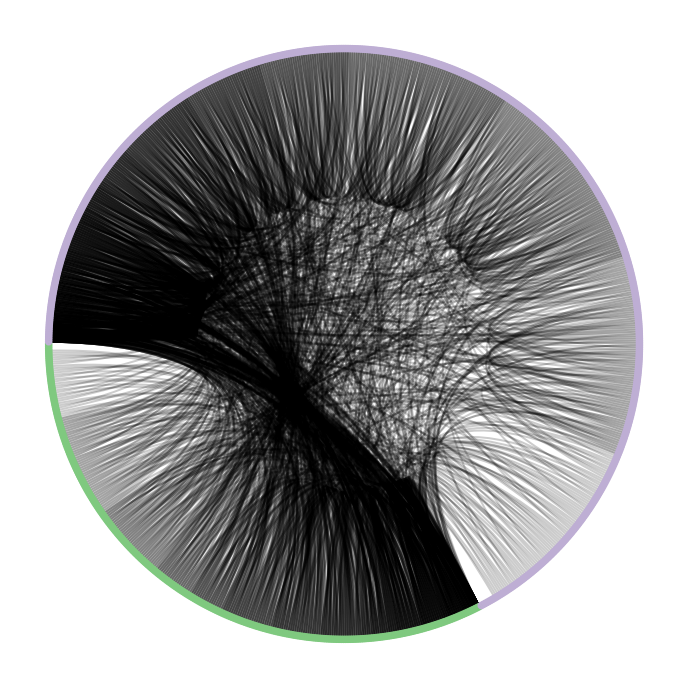In :
import networkx as nx
from custom import load_data as cf
from networkx.algorithms import bipartite
from nxviz import CircosPlot
import numpy as np
import matplotlib.pyplot as plt

%matplotlib inline
%config InlineBackend.figure_format = 'retina'


# Introduction¶

Bipartite graphs are graphs that have two (bi-) partitions (-partite) of nodes. Nodes within each partition are not allowed to be connected to one another; rather, they can only be connected to nodes in the other partition.

Bipartite graphs can be useful for modelling relations between two sets of entities. We will explore the construction and analysis of bipartite graphs here.Let's load a crime data bipartite graph and quickly explore it.

This bipartite network contains persons who appeared in at least one crime case as either a suspect, a victim, a witness or both a suspect and victim at the same time. A left node represents a person and a right node represents a crime. An edge between two nodes shows that the left node was involved in the crime represented by the right node.

In :
G = cf.load_crime_network()
list(G.edges(data=True))[0:5]

Out:
[('p1', 'c1', {'role': 'Suspect'}),
('p1', 'c2', {'role': 'Victim'}),
('p1', 'c3', {'role': 'Victim'}),
('p1', 'c4', {'role': 'Suspect'}),
('c1', 'p756', {'role': 'Suspect'})]
In :
list(G.nodes(data=True))[0:10]

Out:
[('p1', {'bipartite': 'person', 'gender': 1}),
('c1', {'bipartite': 'crime'}),
('c2', {'bipartite': 'crime'}),
('c3', {'bipartite': 'crime'}),
('c4', {'bipartite': 'crime'}),
('p2', {'bipartite': 'person', 'gender': 1}),
('c5', {'bipartite': 'crime'}),
('c6', {'bipartite': 'crime'}),
('c7', {'bipartite': 'crime'}),
('c8', {'bipartite': 'crime'})]

# Projections¶

Bipartite graphs can be projected down to one of the projections. For example, we can generate a person-person graph from the person-crime graph, by declaring that two nodes that share a crime node are in fact joined by an edge.## Exercise¶

Find the bipartite projection function in the NetworkX bipartite module docs, and use it to obtain the unipartite projection of the bipartite graph. (5 min.)

In :
person_nodes = [n for n in G.nodes() if G.node[n]['bipartite'] == 'person']
pG = bipartite.projection.projected_graph(G, person_nodes)
list(pG.nodes(data=True))[0:5]

Out:
[('p1', {'bipartite': 'person', 'gender': 1}),
('p2', {'bipartite': 'person', 'gender': 1}),
('p3', {'bipartite': 'person', 'gender': 1}),
('p4', {'bipartite': 'person', 'gender': 1}),
('p5', {'bipartite': 'person', 'gender': 1})]

## Exercise¶

Try visualizing the person-person crime network by using a Circos plot. Ensure that the nodes are grouped by gender and then by number of connections. (5 min.)

Again, recapping the Circos Plot API:

c = CircosPlot(graph_object, node_color='metadata_key1', node_grouping='metadata_key2', node_order='metadat_key3')
c.draw()
plt.show()  # or plt.savefig('...')

In :
for n, d in pG.nodes(data=True):
pG.node[n]['connectivity'] = len(list(pG.neighbors(n)))
c = CircosPlot(pG, node_color='gender', node_grouping='gender', node_order='connectivity')
c.draw()
plt.savefig('images/crime-person.png', dpi=300)## Exercise¶

Use a similar logic to extract crime links. (2 min.)

In :
crime_nodes = [n for n in G.nodes() if G.node[n]['bipartite'] == 'crime']
cG = bipartite.projection.projected_graph(G, crime_nodes)


## Exercise¶

Can you plot how the crimes are connected, using a Circos plot? Try ordering it by number of connections. (5 min.)

In :
for n in cG.nodes():
cG.node[n]['connectivity'] = float(len(list(cG.neighbors(n))))
c = CircosPlot(cG, node_order='connectivity', node_color='connectivity')
c.draw()
plt.savefig('images/crime-crime.png', dpi=300)